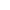# 传奇普通人形怪物设置

1.在怪物DB中Racelme设为0或256的倍数.例如256,512,1024 等.
2.怪物穿什么装备,在Appr设置.具体如下,查看某服装的Shape值,例如 轻型盔甲 的Shape值为2. 则 Appr的值为 1024(即2*512) ～ 1536 (即3*512) 是穿轻型盔甲的怪物,其中前一半1024～1280为男装,后一半1281～1536为女装.其中每段的前2个分别与后面不同发型,例如1024,1025,分别光头,短发两种男发型.1281,1282分别两种女发型.
3.拿的武器类型.设置MP值.本人测试为:设置MP值为(2*武器的SHAPE值)～ (2*武器的SHAPE值+1). 例如:空手为0或1,拿木剑(SHAPE=1)为2-3,铁剑(SHAPE=2)为4-5,屠龙(SHAPE=26)为52
shap 值 计算公式：appy= 500*A+B

B为100，200，300，400，500之间的一个数，
100-300是男的，
400-500是女的，（呵呵 重男轻女哦）

A=6
appy= 500*A+B =500*6+100=3100 （男）
appy= 500*A+B =500*6+200=3200（男）
appy= 500*A+B =500*6+300=3300（男）
appy= 500*A+B =500*6+400=3400（女）
appy= 500*A+B =500*6+500=3500（女）

appy= 500*A+B =500*7+100=3600（男）
appy= 500*A+B =500*7+400=3900（女）
A=8 （道尊）

A=41（翅膀最新衣服）
appy= 500*A+B =500*41+100=20600（男带翅膀新衣服的）

--------------------------------------------------------------------------------

1700 男战 | 1900 女战 3200 天魔神甲 | 3400 圣战宝甲
2200 男法 | 2400 女法 3600 法神披风 | 4000 霓裳羽衣
2600 男道 | 3000 女道 4300 天尊道袍 | 4600 天师长袍
--------------------------------------------------------------------------------

appr 600 race 11

--------------------------------------------------------------------------------